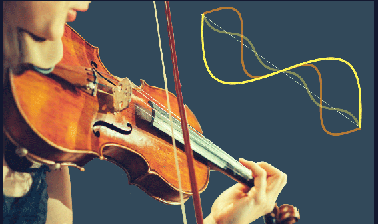# Differential Equations: Fourier Series and Partial Differential Equations

Learn to use Fourier series to solve differential equations with periodic input signals and to solve boundary value problems involving the heat equation and wave equation.## There is one session available:

After a course session ends, it will be archived.
Estimated 11 weeks
5–8 hours per week
Instructor-paced
Instructor-led on a course schedule

Differential equations are the mathematical language we use to describe the world around us. Many phenomena are not modeled by differential equations, but by partial differential equations depending on more than one independent variable. In this course, we will use Fourier series methods to solve ODEs and separable partial differential equations (PDEs). You will learn how to describe any periodic function using Fourier series, and will be able to use resonance and to determine the behavior of systems with periodic input signals that can be described in terms of Fourier series. This course will use MATLAB to assist computations.

In this course we will explore:

• How to process noisy sound files
• The way a beam bends in response to external forces
• How to design of ovens to create strong but lightweight composites
• The motion of a violin string

The five modules in this seriesare being offered as an XSeries on edX. Please visit theDifferential EquationsXSeries Program Pageto learn more and to enroll in the modules.

### At a glance

• Institution: MITx
• Subject: Math
• Level: Intermediate
• Prerequisites:
• Introduction to Differential Equations (basic)
• some linear algebra preferred

# What you'll learn

Skip What you'll learn
• How to describe periodic functions using Fourier series
• How to solve ODEs with Fourier series input
• How to solve separable PDEs using Fourier series inputs and boundary conditions

# Syllabus

Skip Syllabus

Unit 1: Fourier Series

1. Introduction to Fourier series
2. Fourier series with arbitrary periods
3. Using Fourier series to solve differential equations

Unit 2: Partial Differential Equations

1. Boundary conditions and boundary value problems
2. The heat equation
3. The wave equation